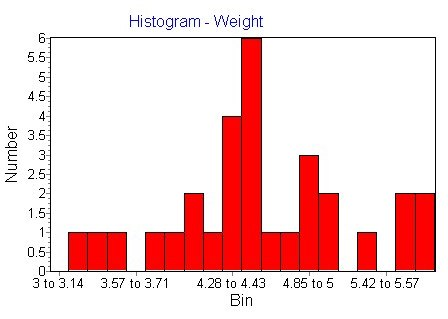Single sample tests: get to the heart of your data

QED Statistics has all the methods you need to examine the basics of your data set:

• Mean - to calculate the arithmetic mean of a list of numbers.
• Median - to calculate the median of a list of numbers.
• Variance - to calculate the variance of a list of numbers.
• Standard deviation - to calculate the standard deviation of a list of numbers.
• Skewness - to calculate the skew of a list of numbers.
• Kurtosis - to calculate the kurtosis (degree of peak) of a list of numbers.
• Probability Plot - to examine the cumulative frequency distribution and investigate normality.
• Box and Whisker - To create a box and whisker plot for a variable.
• Testing Normality - Use Chi, Shapiro-Wilk or Lilliefors tests to test if the variable is normally distributed.
• t-Test - to test the mean of the variable for significant difference from a defined value.
• z Test - to test the mean of the variable for significant difference from a defined value.
• Summary of Data - the data set statistics.Don't forget, for most calculations, Expand & Explore will
show you exactly how the calculation is performed.

Meanwhile, the infinitely customisable charts will emphasise